# Telangana SSC Board Question Paper for Class 10th General Science Part 1 2017 In PDF

## Telangana 10th Standard General Science Part 1 Exam Question Paper 2017 with Solutions – Free Download

Telangana Board Class 10 General Science Part 1 2017 Question Paper with Solutions is the basis for most of the important questions asked, usually in the General Science Part I Physical Sciences exams. Students can download the PDF formats of the solved or unsolved question paper, as per preference, with just a click on the links that are listed in the article. For the students’ convenience, we have also mentioned below in this article the interactive PDF links, from where they can get the printable format of the question papers. The questions and answers are also published on the web-page.

Those students who have cracked the complete complex Physical Sciences topics will find it easy to ace the board exams. They can get acquainted with the types of questions that are asked in the TS Board SSC Class 10 General Science Part 1 exam with the help of these question paper solutions. Students are also urged to practise hard by solving the previous papers of Telangana Board Class 10 before the exams to gauge their performance and their preparation level for the board exams. Referring to these solutions beforehand will help the students identify the mistakes and take steps to avoid the same for the board exams. Solving these paper solutions is the best way for the students to get an overview of the question paper format and the marks’ distribution per section. These question paper solutions also help them to determine the difficulty level of the exams.

### Telangana Board Class 10 General Science Part I 2017 Question Paper with Solutions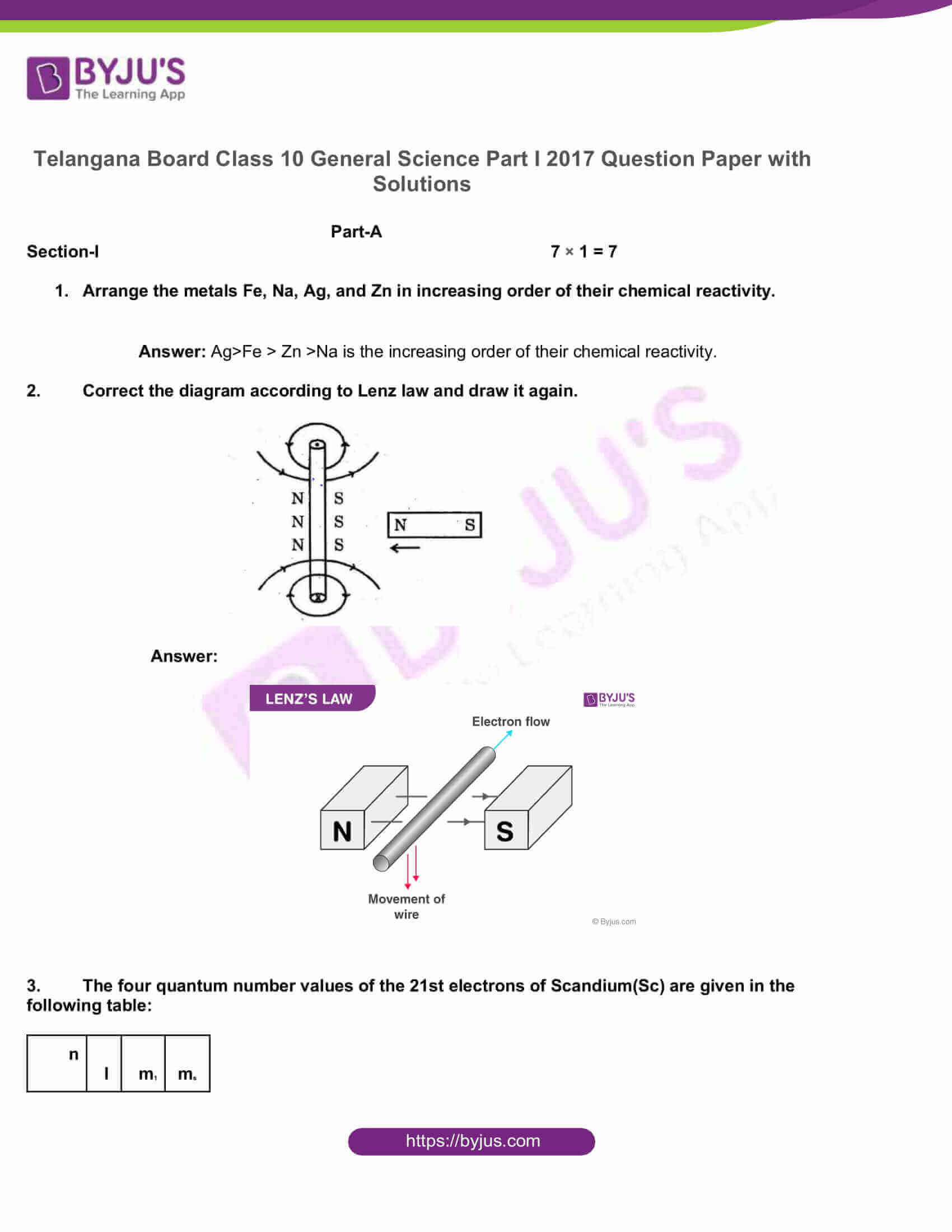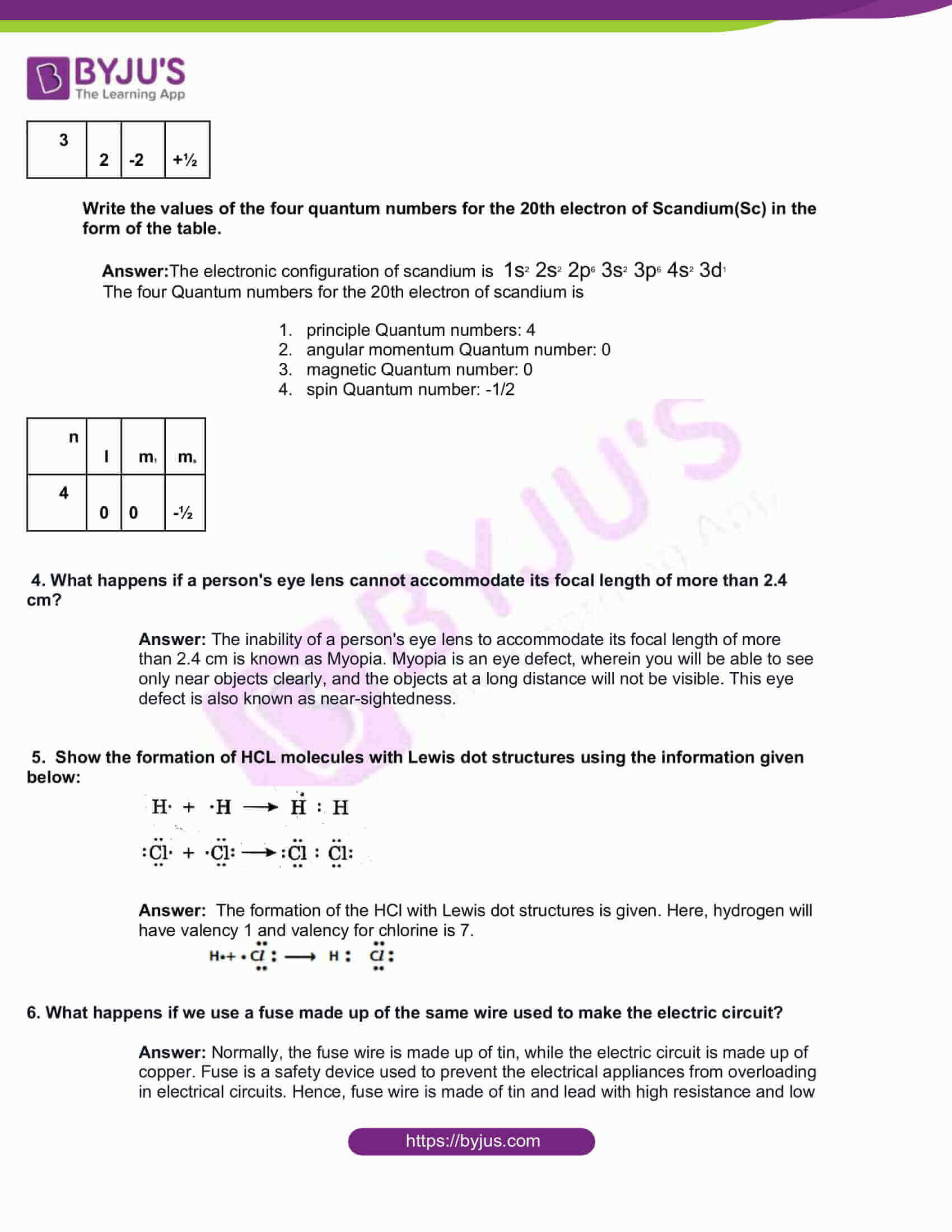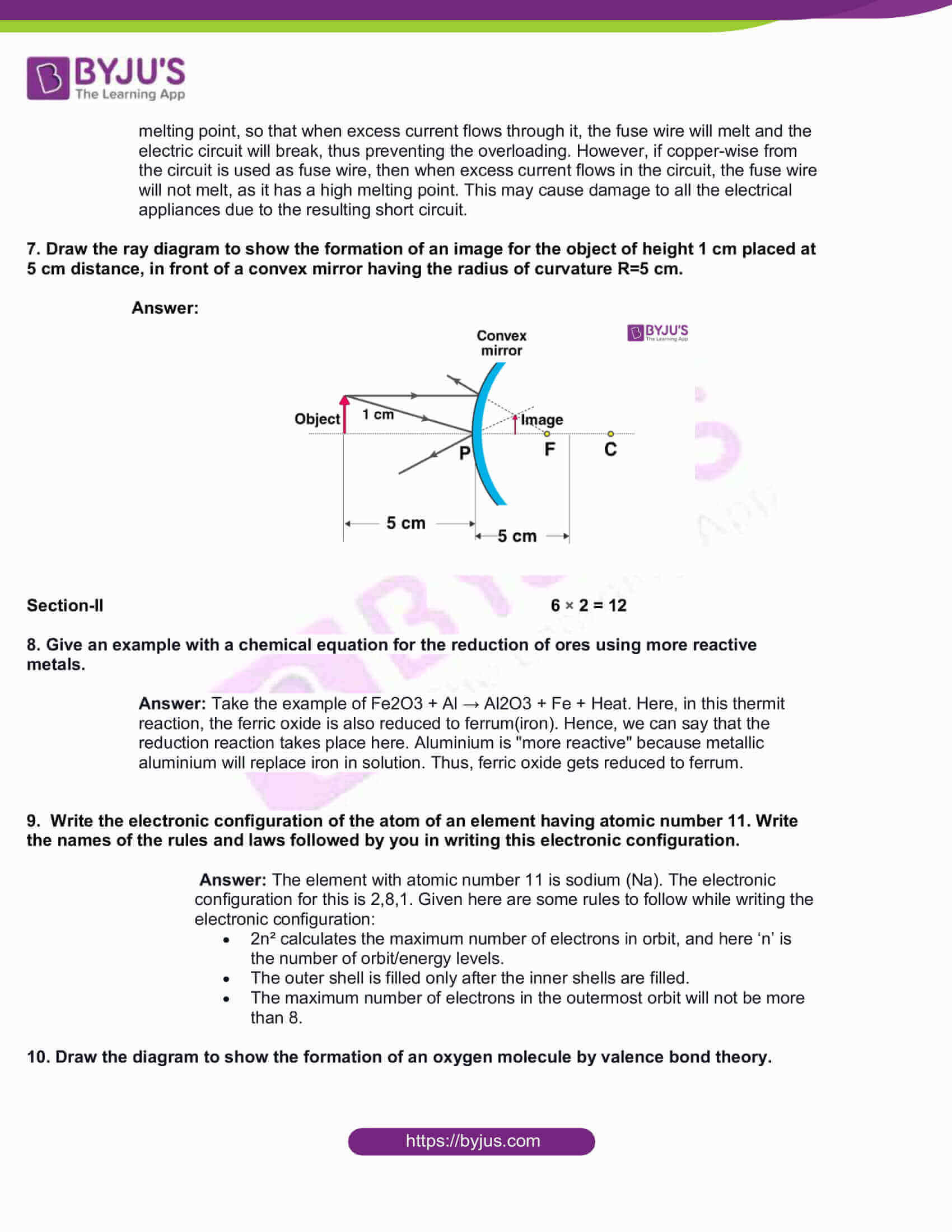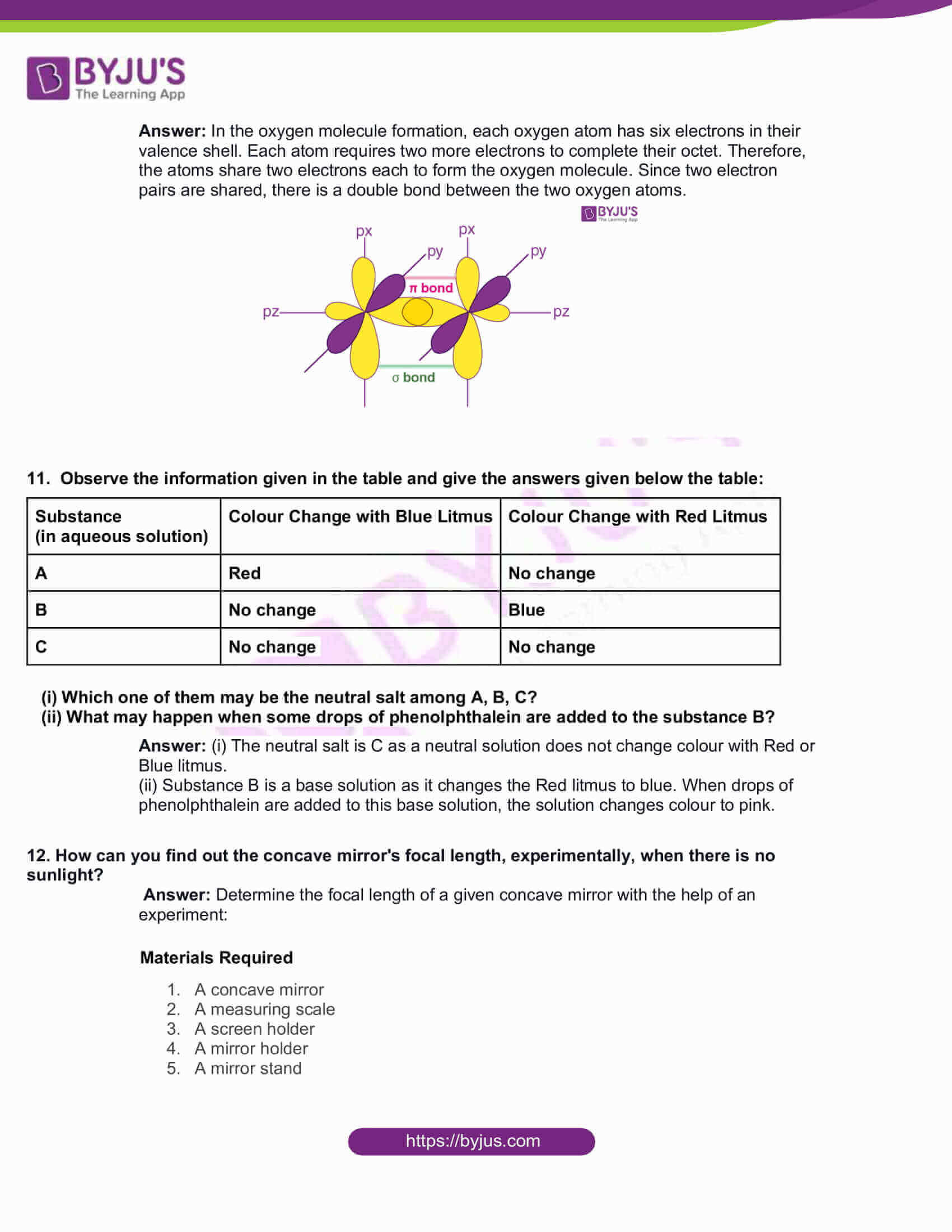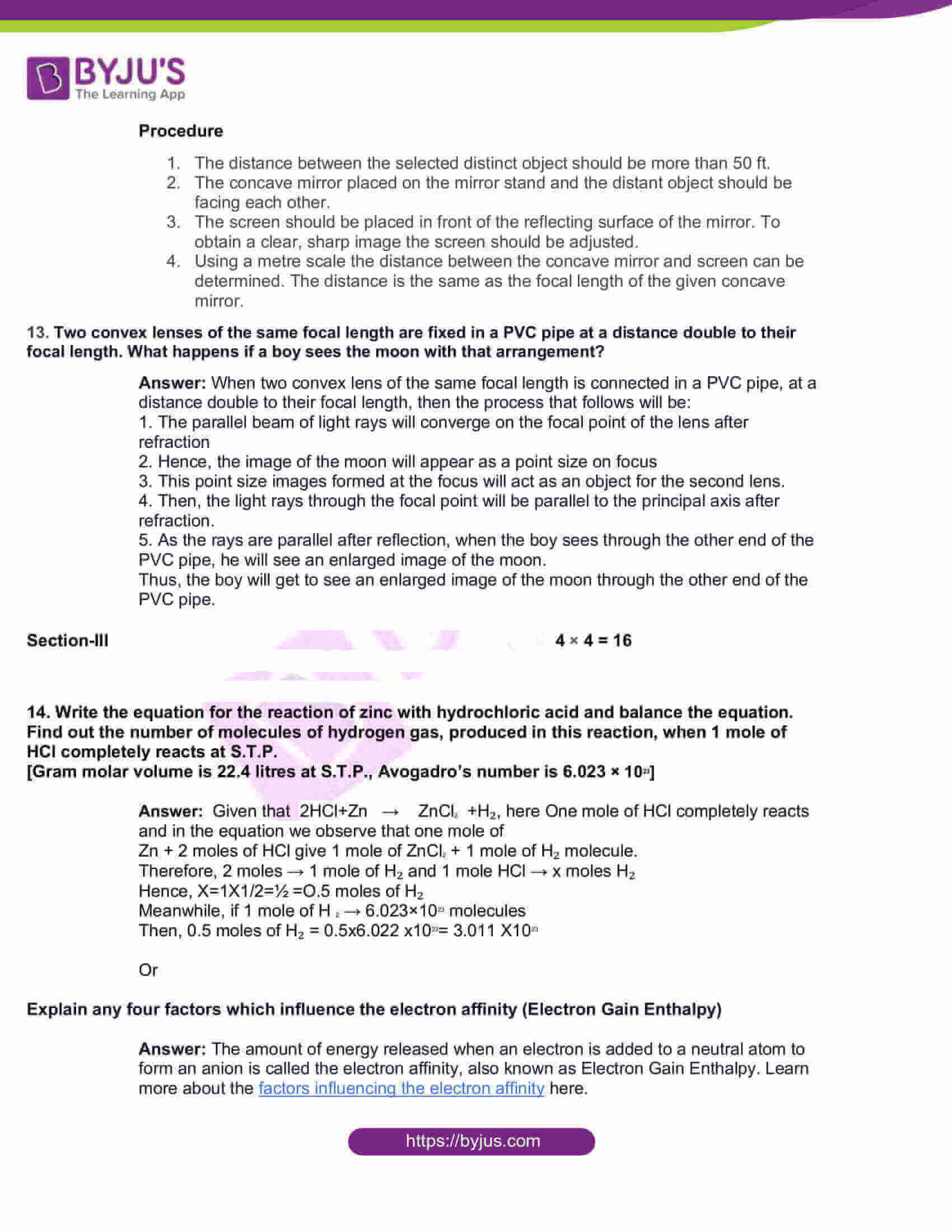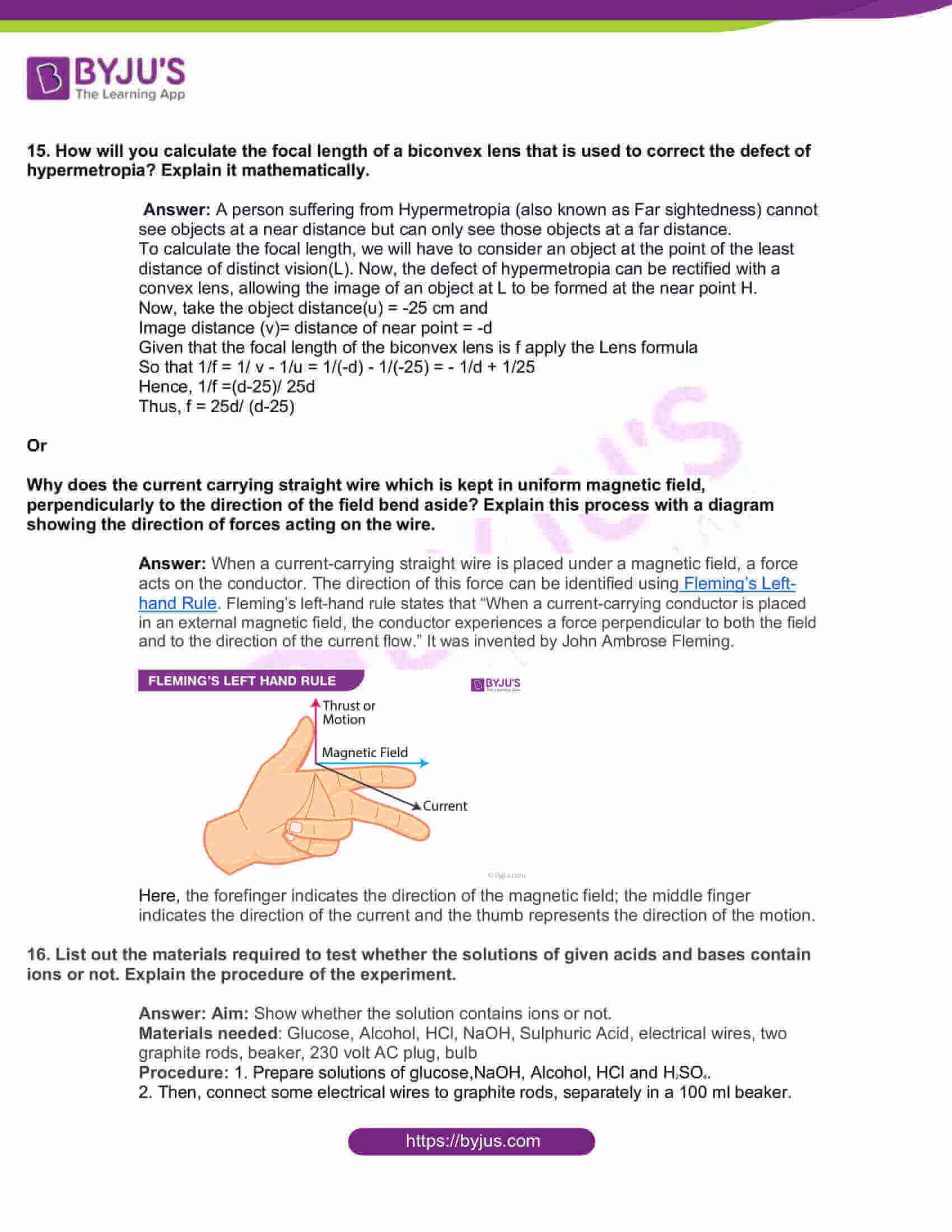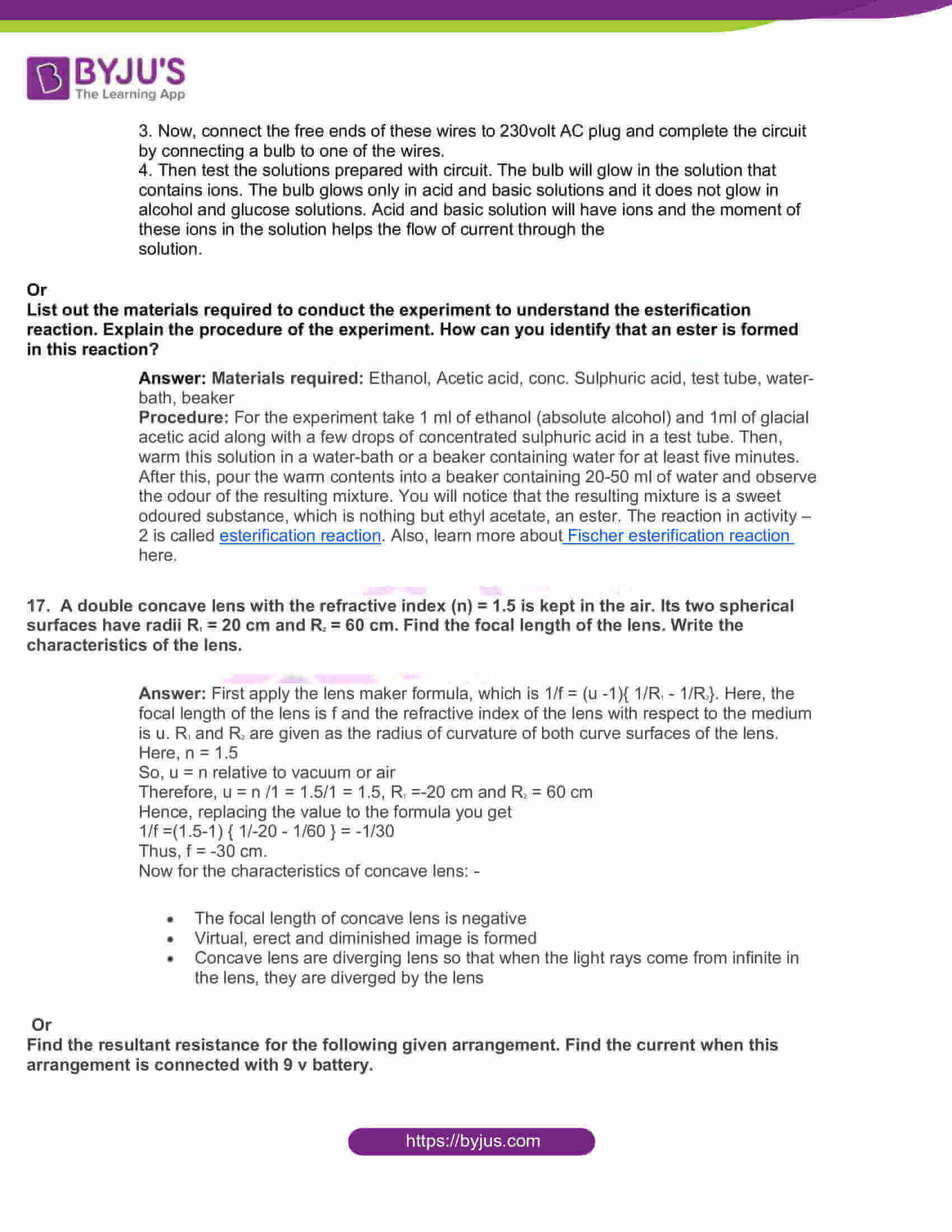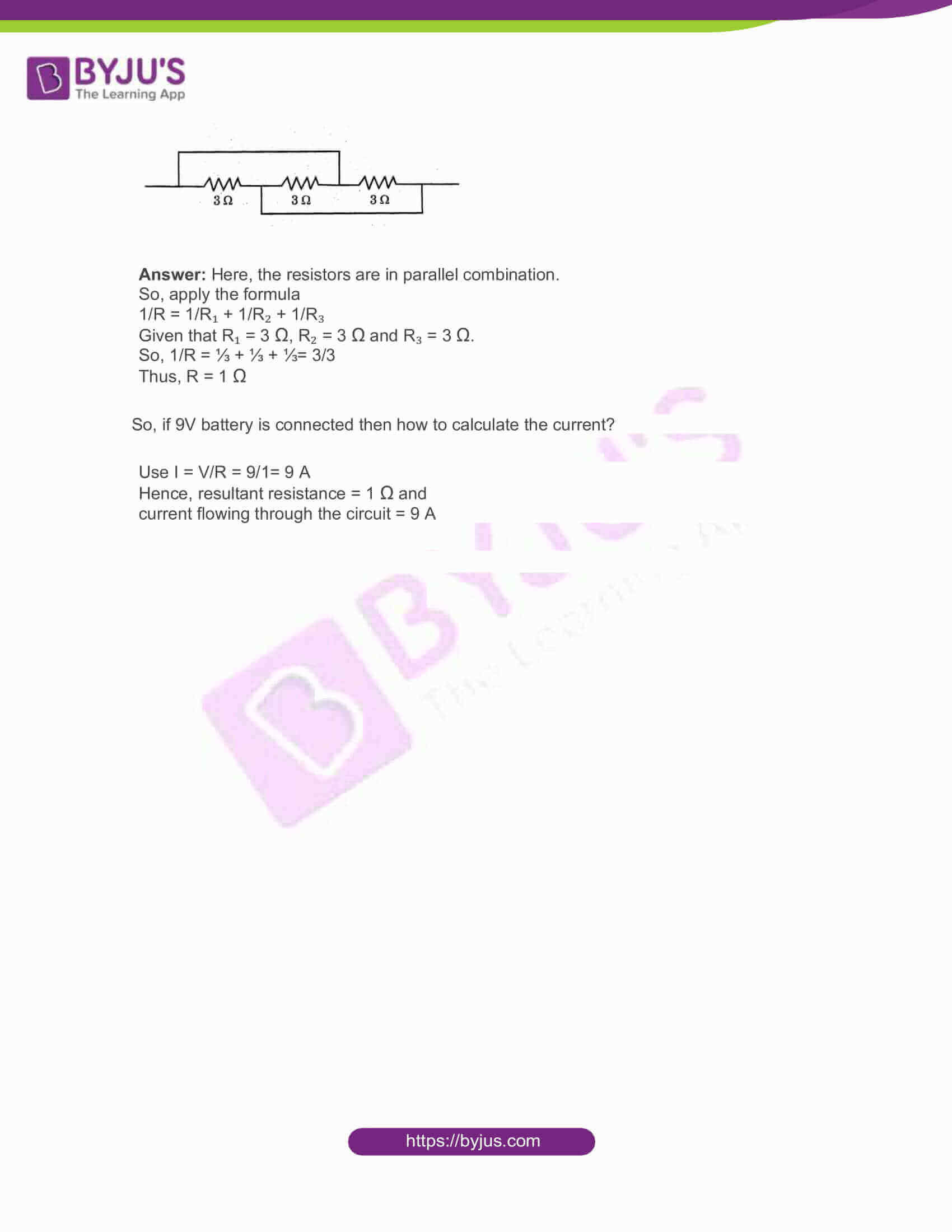Part-A

Section-I 7 × 1 = 7

1. Arrange the metals Fe, Na, Ag, and Zn in increasing order of their chemical reactivity.

Answer: Ag>Fe > Zn >Na is the increasing order of their chemical reactivity.

1. Correct the diagram according to Lenz law and draw it again.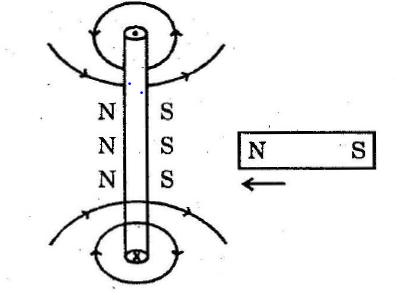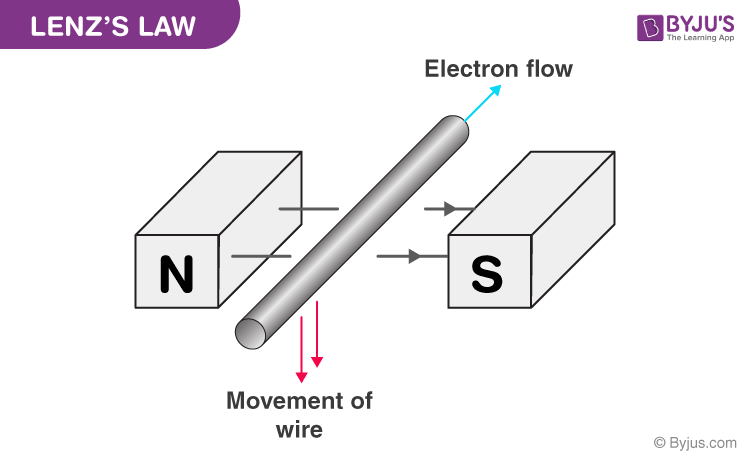1. The four quantum number values of the 21st electrons of Scandium(Sc) are given in the following table:

 n l m1 ms 3 2 -2 +½

Write the values of the four quantum numbers for the 20th electron of Scandium(Sc) in the form of the table.

Answer:The electronic configuration of scandium is 1s2 2s2 2p6 3s2 3p6 4s2 3d1

The four Quantum numbers for the 20th electron of scandium is

1. principle Quantum numbers: 4
2. angular momentum Quantum number: 0
3. magnetic Quantum number: 0
4. spin Quantum number: -1/2
 n l m1 ms 4 0 0 -½

4. What happens if a person’s eye lens cannot accommodate its focal length of more than 2.4 cm?

Answer: The inability of a person’s eye lens to accommodate its focal length of more than 2.4 cm is known as Myopia. Myopia is an eye defect, wherein you will be able to see only near objects clearly, and the objects at a long distance will not be visible. This eye defect is also known as near-sightedness.

5. Show the formation of HCL molecules with Lewis dot structures using the information given below: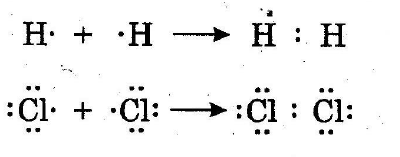Answer: The formation of the HCl with Lewis dot structures is given. Here, hydrogen will have valency 1 and valency for chlorine is 7.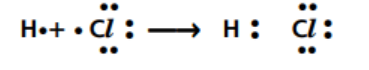6. What happens if we use a fuse made up of the same wire used to make the electric circuit?

Answer: Normally, the fuse wire is made up of tin, while the electric circuit is made up of copper. Fuse is a safety device used to prevent the electrical appliances from overloading in electrical circuits. Hence, fuse wire is made of tin and lead with high resistance and low melting point, so that when excess current flows through it, the fuse wire will melt and the electric circuit will break, thus preventing the overloading. However, if copper-wise from the circuit is used as fuse wire, then when excess current flows in the circuit, the fuse wire will not melt, as it has a high melting point. This may cause damage to all the electrical appliances due to the resulting short circuit.

7. Draw the ray diagram to show the formation of an image for the object of height 1 cm placed at 5 cm distance, in front of a convex mirror having the radius of curvature R=5 cm.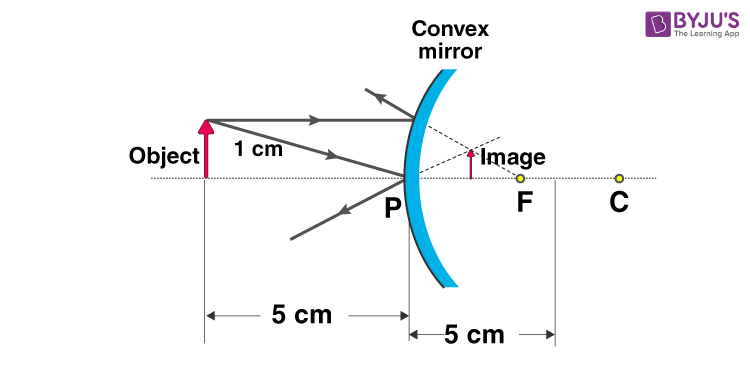Section-II 6 × 2 = 12

8. Give an example with a chemical equation for the reduction of ores using more reactive metals.

Answer: Take the example of Fe2O3 + Al → Al2O3 + Fe + Heat. Here, in this thermit reaction, the ferric oxide is also reduced to ferrum(iron). Hence, we can say that the reduction reaction takes place here. Aluminium is “more reactive” because metallic aluminium will replace iron in solution. Thus, ferric oxide gets reduced to ferrum.

9. Write the electronic configuration of the atom of an element having atomic number 11. Write the names of the rules and laws followed by you in writing this electronic configuration.

Answer: The element with atomic number 11 is sodium (Na). The electronic configuration for this is 2,8,1. Given here are some rules to follow while writing the electronic configuration:

• 2n² calculates the maximum number of electrons in orbit, and here ‘n’ is the number of orbit/energy levels.
• The outer shell is filled only after the inner shells are filled.
• The maximum number of electrons in the outermost orbit will not be more than 8.

10. Draw the diagram to show the formation of an oxygen molecule by valence bond theory.

Answer: In the oxygen molecule formation, each oxygen atom has six electrons in their valence shell. Each atom requires two more electrons to complete their octet. Therefore, the atoms share two electrons each to form the oxygen molecule. Since two electron pairs are shared, there is a double bond between the two oxygen atoms.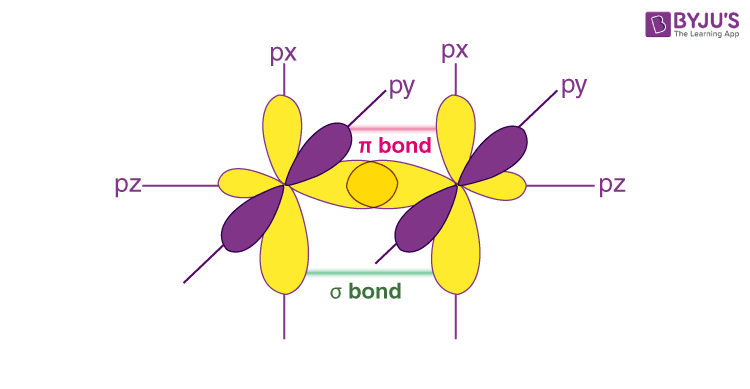11. Observe the information given in the table and give the answers given below the table:

 Substance (in aqueous solution) Colour Change with Blue Litmus Colour Change with Red Litmus A Red No change B No change Blue C No change No change

(i) Which one of them may be the neutral salt among A, B, C?

(ii) What may happen when some drops of phenolphthalein are added to the substance B?

Answer: (i) The neutral salt is C as a neutral solution does not change colour with Red or Blue litmus.

(ii) Substance B is a base solution as it changes the Red litmus to blue. When drops of phenolphthalein are added to this base solution, the solution changes colour to pink.

12. How can you find out the concave mirror’s focal length, experimentally, when there is no sunlight?

Answer: Determine the focal length of a given concave mirror with the help of an experiment:

### Materials Required

1. A concave mirror
2. A measuring scale
3. A screen holder
4. A mirror holder
5. A mirror stand

### Procedure

1. The distance between the selected distinct object should be more than 50 ft.
2. The concave mirror placed on the mirror stand and the distant object should be facing each other.
3. The screen should be placed in front of the reflecting surface of the mirror. To obtain a clear, sharp image the screen should be adjusted.
4. Using a metre scale the distance between the concave mirror and screen can be determined. The distance is the same as the focal length of the given concave mirror.

13. Two convex lenses of the same focal length are fixed in a PVC pipe at a distance double to their focal length. What happens if a boy sees the moon with that arrangement?

Answer: When two convex lens of the same focal length is connected in a PVC pipe, at a distance double to their focal length, then the process that follows will be:

1. The parallel beam of light rays will converge on the focal point of the lens after refraction

2. Hence, the image of the moon will appear as a point size on focus

3. This point size images formed at the focus will act as an object for the second lens.

4. Then, the light rays through the focal point will be parallel to the principal axis after refraction.

5. As the rays are parallel after reflection, when the boy sees through the other end of the PVC pipe, he will see an enlarged image of the moon.

Thus, the boy will get to see an enlarged image of the moon through the other end of the PVC pipe.

Section-III 4 × 4 = 16

14. Write the equation for the reaction of zinc with hydrochloric acid and balance the equation. Find out the number of molecules of hydrogen gas, produced in this reaction, when 1 mole of HCl completely reacts at S.T.P.

[Gram molar volume is 22.4 litres at S.T.P., Avogadro’s number is 6.023 × 1023]

Answer: Given that 2HCl+Zn → ZnCl2 +H₂, here One mole of HCl completely reacts and in the equation we observe that one mole of

Zn + 2 moles of HCl give 1 mole of ZnCl2 + 1 mole of H₂ molecule.

Therefore, 2 moles → 1 mole of H₂ and 1 mole HCl → x moles H₂

Hence, X=1X1/2=½ =O.5 moles of H₂

Meanwhile, if 1 mole of H 2 → 6.023×1023 molecules

Then, 0.5 moles of H₂ = 0.5×6.022 x1023= 3.011 X1023

Or

Explain any four factors which influence the electron affinity (Electron Gain Enthalpy)

Answer: The amount of energy released when an electron is added to a neutral atom to form an anion is called the electron affinity, also known as Electron Gain Enthalpy. Learn more about the factors influencing the electron affinity here.

15. How will you calculate the focal length of a biconvex lens that is used to correct the defect of hypermetropia? Explain it mathematically.

Answer: A person suffering from Hypermetropia (also known as Far sightedness) cannot see objects at a near distance but can only see those objects at a far distance.

To calculate the focal length, we will have to consider an object at the point of the least distance of distinct vision(L). Now, the defect of hypermetropia can be rectified with a convex lens, allowing the image of an object at L to be formed at the near point H.

Now, take the object distance(u) = -25 cm and

Image distance (v)= distance of near point = -d

Given that the focal length of the biconvex lens is f apply the Lens formula

So that 1/f = 1/ v – 1/u = 1/(-d) – 1/(-25) = – 1/d + 1/25

Hence, 1/f =(d-25)/ 25d

Thus, f = 25d/ (d-25)

Or

Why does the current carrying straight wire which is kept in uniform magnetic field, perpendicularly to the direction of the field bend aside? Explain this process with a diagram showing the direction of forces acting on the wire.

Answer: When a current-carrying straight wire is placed under a magnetic field, a force acts on the conductor. The direction of this force can be identified using Fleming’s Left-hand Rule. Fleming’s left-hand rule states that “When a current-carrying conductor is placed in an external magnetic field, the conductor experiences a force perpendicular to both the field and to the direction of the current flow.” It was invented by John Ambrose Fleming.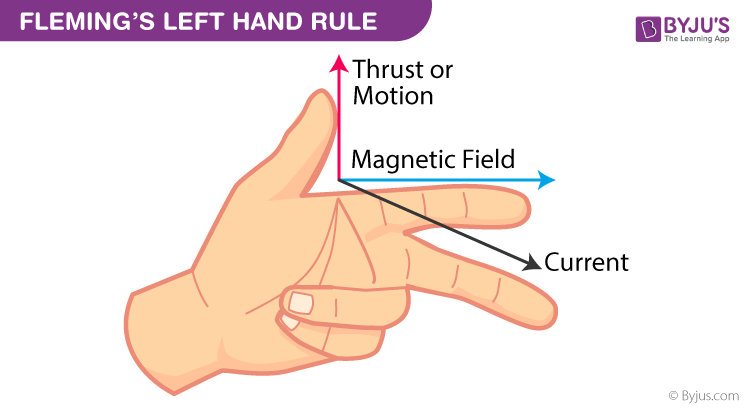Here, the forefinger indicates the direction of the magnetic field; the middle finger indicates the direction of the current and the thumb represents the direction of the motion.

16. List out the materials required to test whether the solutions of given acids and bases contain ions or not. Explain the procedure of the experiment.

Answer: Aim: Show whether the solution contains ions or not.

Materials needed: Glucose, Alcohol, HCl, NaOH, Sulphuric Acid, electrical wires, two graphite rods, beaker, 230 volt AC plug, bulb

Procedure: 1. Prepare solutions of glucose,NaOH, Alcohol, HCl and H2SO4.

2. Then, connect some electrical wires to graphite rods, separately in a 100 ml beaker.

3. Now, connect the free ends of these wires to 230volt AC plug and complete the circuit by connecting a bulb to one of the wires.

4. Then test the solutions prepared with circuit. The bulb will glow in the solution that contains ions. The bulb glows only in acid and basic solutions and it does not glow in alcohol and glucose solutions. Acid and basic solution will have ions and the moment of these ions in the solution helps the flow of current through the solution.

Or

List out the materials required to conduct the experiment to understand the esterification reaction. Explain the procedure of the experiment. How can you identify that an ester is formed in this reaction?

Answer: Materials required: Ethanol, Acetic acid, conc. Sulphuric acid, test tube, water-bath, beaker

Procedure: For the experiment take 1 ml of ethanol (absolute alcohol) and 1ml of glacial acetic acid along with a few drops of concentrated sulphuric acid in a test tube. Then, warm this solution in a water-bath or a beaker containing water for at least five minutes. After this, pour the warm contents into a beaker containing 20-50 ml of water and observe the odour of the resulting mixture. You will notice that the resulting mixture is a sweet odoured substance, which is nothing but ethyl acetate, an ester. The reaction in activity – 2 is called esterification reaction. Also, learn more about Fischer esterification reaction here.

17. A double concave lens with the refractive index (n) = 1.5 is kept in the air. Its two spherical surfaces have radii R1 = 20 cm and R2 = 60 cm. Find the focal length of the lens. Write the characteristics of the lens.

Answer: First apply the lens maker formula, which is 1/f = (u -1){ 1/R1 – 1/R2}. Here, the focal length of the lens is f and the refractive index of the lens with respect to the medium is u. R1 and R2 are given as the radius of curvature of both curve surfaces of the lens.

Here, n = 1.5

So, u = n relative to vacuum or air

Therefore, u = n /1 = 1.5/1 = 1.5 , R1 =-20 cm and R2 = 60 cm

Hence, replacing the value to the formula you get

1/f =(1.5-1) { 1/-20 – 1/60 } = -1/30

Thus, f = -30 cm.

Now for the characteristics of concave lens:-

• The focal length of concave lens is negative
• Virtual, erect and diminished image is formed
• Concave lens are diverging lens so that when the light rays come from infinite in the lens, they are diverged by the lens

Or

Find the resultant resistance for the following given arrangement. Find the current when this arrangement is connected with 9 v battery.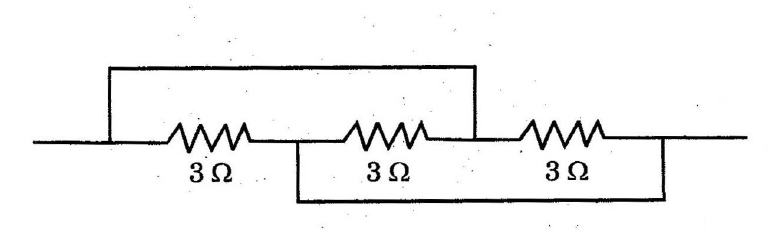Answer: Here, the resistors are in parallel combination.

So apply the formula

1/R = 1/R₁ + 1/R₂ + 1/R₃

Given that R₁ = 3 Ω, R₂ = 3 Ω and R₃ = 3 Ω.

So, 1/R = ⅓ + ⅓ + ⅓= 3/3

Thus, R = 1 Ω

So, if 9V battery is connected then how to calculate the current?

Use I = V/R = 9/1= 9 A

Hence, resultant resistance = 1 Ω and

current flowing through the circuit = 9 A